The OpenFOAM FoundationRepresentation of a 3D Cartesian coordinate system as a Vector of vectors. More...

Inheritance diagram for triad: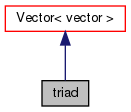[legend]
Collaboration diagram for triad: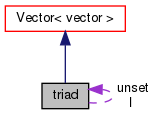[legend]

## Public Member Functions

Construct null. More...

triad (const Vector< vector > &vv)
Construct from components. More...

triad (const vector &x, const vector &y, const vector &z)
Construct from coordinate axes. More...

triad (const vector &pa)
Construct from a primary axis with the other two unset. More...

triad (const quaternion &q)
Construct from a quaternion. More...

triad (const tensor &t)
Construct from a tensor. More...

Construct from Istream. More...

bool set (const direction d) const
Is the vector in the direction d set. More...

bool set () const
Are all the vector set. More...

void orthogonalize ()
Orthogonalize this triad so that it is ortho-normal. More...

void normalize ()
Normalize each set axis vector to have a unit magnitude. More...

void align (const vector &v)
Align this triad with the given vector v. More...

triad sortxyz () const
Sort the axes such that they are closest to the x, y and z axes. More...

operator quaternion () const
Convert to a quaternion. More...

void operator= (const Vector< vector > &)

void operator= (const tensor &t)

void operator+= (const triad &t2)Public Member Functions inherited from Vector< vector >
Vector ()
Construct null. More...

Vector (const Foam::zero)
Construct initialized to zero. More...

Vector (const VectorSpace< Vector< Cmpt2 >, Cmpt2, 3 > &)
Construct given VectorSpace of the same rank. More...

Vector (const vector &vx, const vector &vy, const vector &vz)
Construct given three components. More...

Vector (Istream &)
Construct from Istream. More...

const vectorx () const

vectorx ()

const vectory () const

vectory ()

const vectorz () const

vectorz ()

const Vector< vector > & centre (const Foam::List< Vector< vector >> &) const
Return *this (used for point which is a typedef to Vector<scalar>. More...Public Member Functions inherited from VectorSpace< Vector< vector >, vector, 3 >
VectorSpace ()
Construct null. More...

VectorSpace (const Foam::zero)
Construct initialized to zero. More...

VectorSpace (Istream &)
Construct from Istream. More...

VectorSpace (const VectorSpace< Form2, Cmpt2, Ncmpts > &)
Construct as copy of a VectorSpace with the same size. More...

const vectorcomponent (const direction) const

vectorcomponent (const direction)

void component (vector &, const direction) const

void replace (const direction, const vector &)

const ConstBlock< SubVector, BStart > block () const

const VectorSpace< Vector< vector >, vector, Ncmpts >::template ConstBlock< SubVector, BStart > block () const

const vectoroperator[] (const direction) const

vectoroperator[] (const direction)

void operator+= (const VectorSpace< Vector< vector >, vector, Ncmpts > &)

void operator-= (const VectorSpace< Vector< vector >, vector, Ncmpts > &)

void operator= (const Foam::zero)

void operator*= (const scalar)

void operator/= (const scalar)

## Static Public Member Functions

static direction primaryDirection (const vector &v)
Return the primary direction of the vector v. More...

static vector orthogonal (const vector &v1, const vector &v2)
Return the vector orthogonal to the two provided. More...Static Public Member Functions inherited from VectorSpace< Vector< vector >, vector, 3 >
static direction size ()
Return the number of elements in the VectorSpace = Ncmpts. More...

static Vector< vectoruniform (const vector &s)
Return a VectorSpace with all elements = s. More...

## Static Public Attributes

static const triad I

static const triad unsetStatic Public Attributes inherited from Vector< vector >
static const direction rank
Rank of Vector is 1. More...Static Public Attributes inherited from VectorSpace< Vector< vector >, vector, 3 >
static const direction dim
Dimensionality of space. More...

static const direction nComponents
Number of components in this vector space. More...

static const direction mRows

static const direction nCols

static const char *const typeName

static const char *const componentNames []

static const Vector< vectorzero

static const Vector< vectorone

static const Vector< vectormax

static const Vector< vectormin

static const Vector< vectorrootMax

static const Vector< vectorrootMin

## Friends

Istreamoperator>> (Istream &, triad &)

Ostreamoperator<< (Ostream &, const triad &)

## Additional Inherited MembersPublic Types inherited from Vector< vector >
enum  components
Component labeling enumeration. More...

typedef Vector< labellabelType
Equivalent type of labels used for valid component indexing. More...Public Types inherited from VectorSpace< Vector< vector >, vector, 3 >
typedef VectorSpace< Vector< vector >, vector, Ncmpts > vsType
VectorSpace type. More...

typedef vector cmptType
Component type. More...Public Attributes inherited from VectorSpace< Vector< vector >, vector, 3 >
vector v_ [Ncmpts]
The components of this vector space. More...

## Detailed Description

Representation of a 3D Cartesian coordinate system as a Vector of vectors.

Foam::quaternion
Source files

Definition at line 65 of file triad.H.

## ◆ triad() [1/7]

inline

Construct null.

Definition at line 28 of file triadI.H.

## ◆ triad() [2/7]

 triad ( const Vector< vector > & vv )
inline

Construct from components.

Definition at line 34 of file triadI.H.

## ◆ triad() [3/7]

 triad ( const vector & x, const vector & y, const vector & z )
inline

Construct from coordinate axes.

Definition at line 40 of file triadI.H.

## ◆ triad() [4/7]

 triad ( const vector & pa )
inline

Construct from a primary axis with the other two unset.

Definition at line 46 of file triadI.H.

Here is the call graph for this function: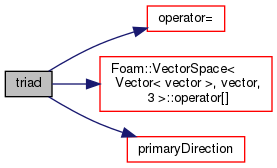## ◆ triad() [5/7]

 triad ( const quaternion & q )

Construct from a quaternion.

Definition at line 89 of file triad.C.

Here is the call graph for this function: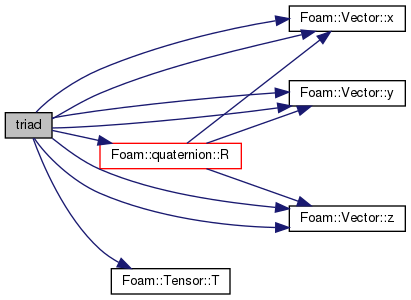## ◆ triad() [6/7]

 triad ( const tensor & t )

Construct from a tensor.

Definition at line 98 of file triad.C.

Here is the call graph for this function: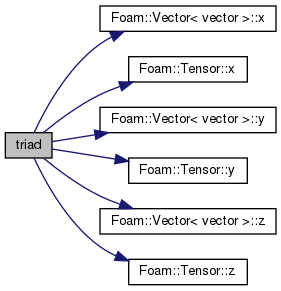## ◆ triad() [7/7]

 triad ( Istream & is )
inline

Construct from Istream.

Definition at line 53 of file triadI.H.

## ◆ set() [1/2]

 bool set ( const direction d ) const
inline

Is the vector in the direction d set.

Definition at line 61 of file triadI.H.

Referenced by triSurface::curvature(), Foam::diff(), and triad::operator+=().

Here is the call graph for this function: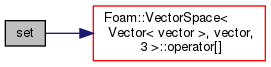Here is the caller graph for this function: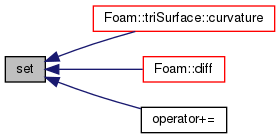## ◆ set() [2/2]

 bool set ( ) const
inline

Are all the vector set.

Definition at line 67 of file triadI.H.

## ◆ primaryDirection()

 Foam::direction primaryDirection ( const vector & v )
inlinestatic

Return the primary direction of the vector v.

Definition at line 73 of file triadI.H.

Here is the call graph for this function: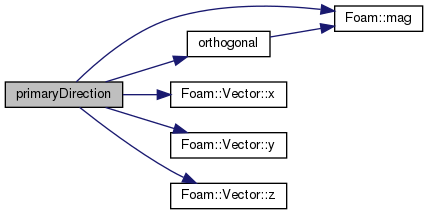Here is the caller graph for this function: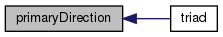## ◆ orthogonal()

 Foam::vector orthogonal ( const vector & v1, const vector & v2 )
inlinestatic

Return the vector orthogonal to the two provided.

Definition at line 91 of file triadI.H.

References Foam::mag(), and triad::unset.

Here is the call graph for this function: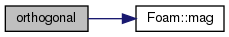Here is the caller graph for this function: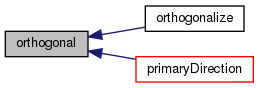## ◆ orthogonalize()

 void orthogonalize ( )

Orthogonalize this triad so that it is ortho-normal.

Definition at line 108 of file triad.C.

Here is the call graph for this function: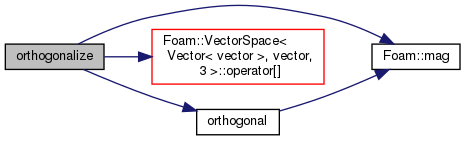## ◆ normalize()

 void normalize ( )
inline

Normalize each set axis vector to have a unit magnitude.

Definition at line 111 of file triadI.H.

Referenced by triSurface::curvature().

Here is the call graph for this function: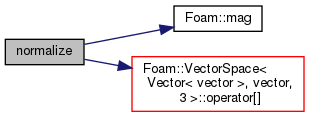Here is the caller graph for this function: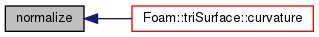## ◆ align()

 void align ( const vector & v )

Align this triad with the given vector v.

by rotating the most aligned axis to be coincident with v

Definition at line 255 of file triad.C.

Here is the call graph for this function: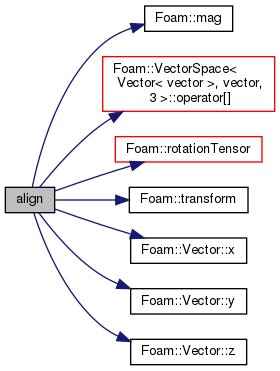## ◆ sortxyz()

 Foam::triad sortxyz ( ) const

Sort the axes such that they are closest to the x, y and z axes.

Definition at line 300 of file triad.C.

Referenced by Foam::diff().

Here is the call graph for this function: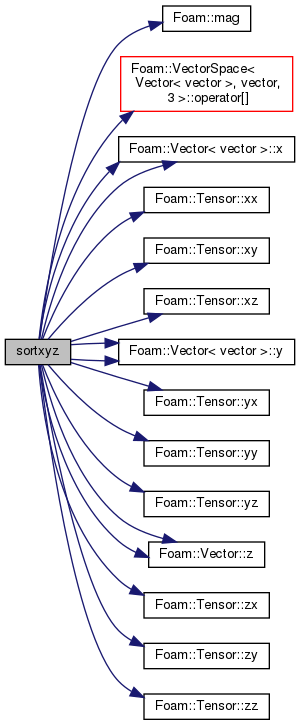Here is the caller graph for this function: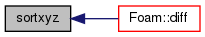## ◆ operator quaternion()

 operator quaternion ( ) const

Convert to a quaternion.

## ◆ operator=() [1/2]

 void operator= ( const Vector< vector > & vv )
inline

Definition at line 121 of file triadI.H.

Here is the call graph for this function: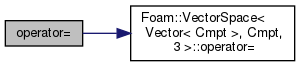Here is the caller graph for this function: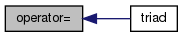## ◆ operator=() [2/2]

 void operator= ( const tensor & t )

Definition at line 393 of file triad.C.

Here is the call graph for this function: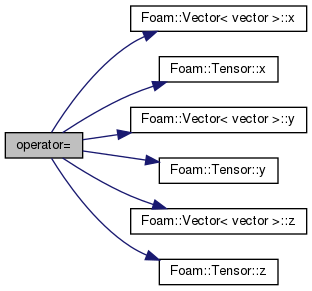## ◆ operator+=()

 void operator+= ( const triad & t2 )

without normalizing or orthogonalizing

Definition at line 192 of file triad.C.

Here is the call graph for this function: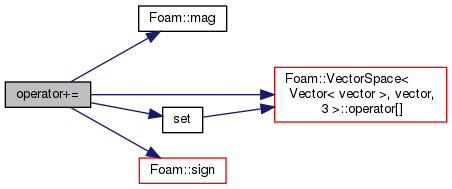## ◆ operator>>

 Istream& operator>> ( Istream & , triad & )
friend

## ◆ operator<<

 Ostream& operator<< ( Ostream & , const triad & )
friend

## ◆ I

static

Definition at line 98 of file triad.H.